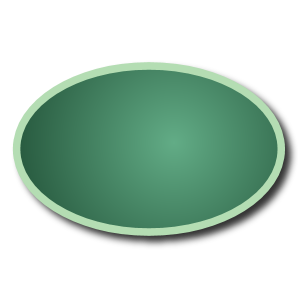Games
Problems
Go Pro!

# Exploring the Equation of an Ellipse

Lesson Plans > Mathematics > Geometry > Analytic Geometry > Conic Sections > Ellipses

## Exploring the Equation of an EllipseHow does the equation of an ellipse relate to its graph?

I Introduce the ellipse concept

"An ellipse looks like an oval. Pretty much a circle that is wider or taller in 1 of its dimensions. You can see pictures

of ellipses here http://www.mathwarehouse.com/ellipse/equation-of-ellipse.php"

II. Exploring the equation and its graph

(To do the following part of the exercise , please open up www.meta-calculator.com, an online graphing calculator that can graph

ellipses. We will explore the way that changing the equation affects the graph of the ellipse)

The general equation of an ellipse looks like x2/a +y2/b = 1 .

Now lets explore the roles of each of these 2 variables. First, let's make b =5 and try exploring different values for 'a'

Graph the following equations, making note of the graph of each:

x2/1 +y2/5 = 1 , a = 1
x2/2 +y2/5 = 1 , a = 2
x2/3 +y2/5 = 1 , a = 3
x2/4 +y2/5 = 1 , a = 4
x2/5 +y2/5 = 1 , a = 5

What effect does increasing 'a'have on the equation ?

Now, take a guess, what do you think will be the effect of changing the variable underneath the y2 term? For instance, if the

term under y2 starts at 1 and then increases, predict how the graph will change:

x2/5 +y2/1 = 1 , b = 1
x2/5 +y2/2 = 1 , b = 2
x2/5 +y2/3 = 1 , b = 3
x2/5 +y2/4 = 1 , b = 4
x2/5 +y2/5 = 1 , b = 5

Concluding question, in terms of 'a' and 'b', what is true when the ellipse becomes a circle?

Lesson by vernonmorris1

# Blogs on This SiteReviews and book lists - books we love!The site administrator fields questions from visitors.Like us on Facebook to get updates about new resources